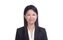cancel
Showing results for
Did you mean:

Fabric is Generally Available. Browse Fabric Presentations. Work towards your Fabric certification with the Cloud Skills Challenge.Resolver IV

## Summarize by one column ignoring other columns

Hello,

I need some help with restructing one of my measures. I am trying to summarize a table by a particular column and calculating the MIN datetime, but I would like to ignore other column. Here is the example of what I am trying to do.

Actual Data

 Level Customer Join Date filterRecords Basic A 1/31/2022 10:15 1 Premium A 1/31/2022 10:35 1 Basic B 1/31/2022 10:55 1 Basic B 1/31/2022 10:25 1 Premium B 1/31/2022 11:35 1 Basic A 1/31/2022 10:15 0 Premium A 1/31/2022 10:35 0 Basic C 1/31/2022 10:15 1 Premium C 1/30/2022 10:35 1 Basic D 1/31/2022 10:55 1 Basic D 1/31/2022 10:25 1 Premium D 1/28/2022 11:35 1 Basic C 1/31/2022 10:15 0 Premium C 1/31/2022 10:35 0 Basic E 1/31/2022 10:55 1 Basic E 1/31/2022 10:25 1 Premium E 1/21/2022 11:35 1

This is a subset of how my data looks like. In this (filterRecords) is one of the measure that I have created based on certain criteria.

Below is my desired result

 Basic 2 Premium 3 Total 5

I want to calculate total number of customers based on the condition where filterRecords= 1 and then I need to count the customers based on their minimum join date MIN (joinDate). Currently my measure is returning two records for customer A as there are 2 Levels against customer A.

I would like the measure to return only one record for customer A based on the MIN(joinDate).

Here is the measure I have tried.

customerCount =
var tempTable1 =
CALCULATETABLE(
Table,
FILTER( Table, Table[_filterRecords] = 1 ))
var tempTable2 =
SUMMARIZE(tempTable1,Table[Customer],"Min Date", CALCULATE(MIN(Table[JoinDate]), ALLEXCEPT(Table, Table[Customer])))
RETURN
COUNTROWS(tempTable2)

Can someone please help me with this measure ? I am not sure what I am doing wrong here.

Any help on this would be greatly appreciated.

Thank you

Regards

1 ACCEPTED SOLUTIONSuper User

You were pretty close.

customerCount =
VAR c =
CALCULATETABLE (
'Table',
ALLEXCEPT ( 'Table', 'Table'[Level] ),
'Table'[filterRecords] = 1
-- only consider valid records
VAR j =
c,
"min join",
VAR d = [Customer]
RETURN
CALCULATE ( MIN ( 'Table'[Join Date] ), ALL ( 'Table' ), 'Table'[Customer] = )
-- calculate earliest join date
VAR f =
FILTER ( j, [Join Date] = [min join] -- only consider rows for min join date
RETURN
COUNTROWS ( )

or all in one:

customerCount =
COUNTROWS (
FILTER (
CALCULATETABLE (
'Table',
ALLEXCEPT ( 'Table', 'Table'[Level] ),
'Table'[filterRecords] = 1
),
"min join",
VAR d = [Customer]
RETURN
CALCULATE ( MIN ( 'Table'[Join Date] ), ALL ( 'Table' ), 'Table'[Customer] = )
),
[Join Date] = [min join]
)
)

``````
``````

2 REPLIES 2Resident Rockstar

Hi @JIGAR

When you summarize your temp table on Customer, there is no context of Level...I did add a Calculated column instead``````Check =
VAR T1=FILTER(ALL('Table'),'Table'[filterRecords]=1&&[Customer]=EARLIER('Table'[Customer]))
VAR T2=GROUPBY(T1,[Customer],"minDate",MINX(CURRENTGROUP(),[Join Date]))
RETURN
IF([Join Date]=MAXX(T2,[minDate]),1,0)

customerCount = CALCULATE(COUNTROWS(VALUES('Table'[Customer])),'Table'[Check]=1)``````Super User

You were pretty close.

customerCount =
VAR c =
CALCULATETABLE (
'Table',
ALLEXCEPT ( 'Table', 'Table'[Level] ),
'Table'[filterRecords] = 1
-- only consider valid records
VAR j =
c,
"min join",
VAR d = [Customer]
RETURN
CALCULATE ( MIN ( 'Table'[Join Date] ), ALL ( 'Table' ), 'Table'[Customer] = )
-- calculate earliest join date
VAR f =
FILTER ( j, [Join Date] = [min join] -- only consider rows for min join date
RETURN
COUNTROWS ( )

or all in one:

customerCount =
COUNTROWS (
FILTER (
CALCULATETABLE (
'Table',
ALLEXCEPT ( 'Table', 'Table'[Level] ),
'Table'[filterRecords] = 1
),
"min join",
VAR d = [Customer]
RETURN
CALCULATE ( MIN ( 'Table'[Join Date] ), ALL ( 'Table' ), 'Table'[Customer] = )
),
[Join Date] = [min join]
)
)

``````
``````Announcements#### Power BI Monthly Update - November 2023

Check out the November 2023 Power BI update to learn about new features.#### Fabric Community News unified experience

Read the latest Fabric Community announcements, including updates on Power BI, Synapse, Data Factory and Data Activator.#### The largest Power BI and Fabric virtual conference

130+ sessions, 130+ speakers, Product managers, MVPs, and experts. All about Power BI and Fabric. Attend online or watch the recordings.Top Solution Authors
Top Kudoed Authors
Users online (2,637)